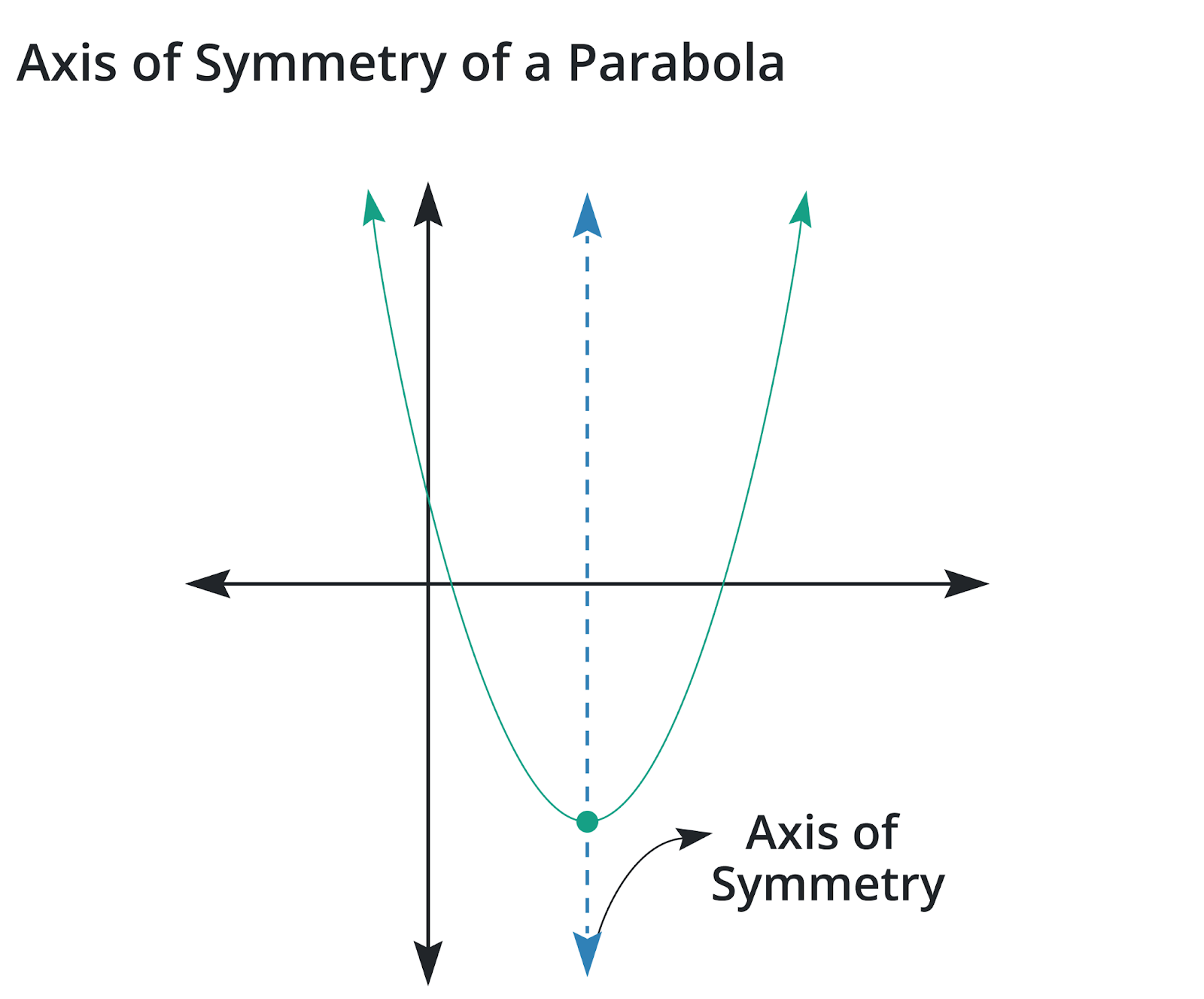# Axis of Symmetry Calculator

To use the Axis of Symmetry Calculator, enter the function in the required input field to calculate the axis of symmetry

Give Us Feedback

## Axis of Symmetry Calculator

Axis of symmetry calculator is an online tool used to evaluate the vertex form of the given function with the help of its formula. This symmetry calculator finds the axis of symmetry of a symmetrical shape (parabola, ellipse, or hyperbola). This calculator provides the result of vertex form with steps.

## What is the Axis of Symmetry?

Axis of symmetry (a.k.a line of symmetry) is a line that divides a symmetrical shape into two congruent parts.### Formula

Formula for a quadratic function f(x) = ax² + bx + c:

x = -b / 2a

Example

Find Axis of symmetry  for f(x) = x² + 4x −1

Solution

Step 1: Take the given function and find the values of a & b.

f(x) = x² + 4x −1

a = 1

b = 4

Step 2: Take the formula for the quadratic function and place the values of a & b in it.

x = -b / 2a

x = -4 / 2(1)

x = -4 / 2

x = -2

### Math Tools